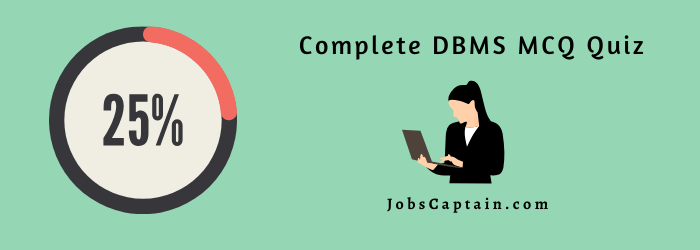# Database Management System MCQ Quiz Set 6Quiz 1 2 3 4 5 6 7

Question 1. Which of three schemas used in three-schema model is a complete logical View of database?

(A) Implementation

(B) Internal

(C) Conceptual

(D) External

(C) Conceptual

Question 2. Which of three schemas used in three-schema model shows physical storage using a particular product or technique?

(A) Conceptual

(B) Internal

(C) External

(D) Implementation

(B) Internal

Question 3. The type of data independence in which the internal schema can be modified without modifying the conceptual schema is classified as

(A) logical data independence

(B) external level independence

(C) physical data independence

(D) conceptual level independence

(C) physical data independence

Question 4. Database __________ which is the logical design of the database, and the database _______ which is a snapshot of the data in the database at a given instant in time.

(A) Instance, Schema

(B) Relation, Domain

(C) Relation, Schema

(D) Schema, Instance

(D) Schema, Instance

Question 5. Relational schemas and other metadata about relations are stored in a structure called the _____?

(A) Data Dictionary

(B) Log

(C) Catalog

(A) Data Dictionary

Question 6. Which of the following enables the user to modify data structures Without affecting existing programs that use them?

(A) Data relationships

(B) Data independence

(C) Data dependence

(D) Data Integration

(B) Data independence

Question 7. Duplicate data in multiple data files is called_____?

(A) Data Integrity

(B) Data multiplication

(C) Data redundancy

(D) None

(C) Data redundancy

Question 8. Commit and rollback are related to ……….?

(A) Data integrity

(B) Data security

(C) Data sharing

(D) Data consistency

(D) Data consistency

Question 9. ……… servers which is widely used in relational database systems.

(A) Data servers

(B) Transaction servers

(C) Query servers

(D) Client servers

(B) Transaction servers

Question 10. A collection of data designed to be used by different people is called a/an __________.

(A) Organization

(B) Relationship

(C) Database

(D) Schema

(C) Database

Question 11. Which of the following is the process of selecting the data storage and data access characteristics of the database?

(A)Logical database design

(B)Evaluation and selecting

(C)Testing and performance tuning

(D)Physical database design

(D)Physical database design

Question 12. Overall logical design of the database is known as _______ ?

(A) Sub Schema

(B) Schema

(C) Instance

(D) Mapping

(B) Schema

Question 13. Actual data or information means _______

(A) Sub Schema

(B) Instance

(C) Mapping

(D) Schema

(B) Instance

Question 14. _____ is the lowest level of the data abstraction.

(A) Internal Level

(B) Conceptual Level

(C) External Level

(D) Data Layer

(A) Internal Level

Question 15. _______ hides details about data types and data-structure used to develop application at logical level.

(A) Conceptual Level

(B) Physical Level

(C) External Level

(D) Internal Level

(C)External Level

Question 16. In external mapping, how many mappings are there between conceptual and external levels ?

(A) 1

(B) 2

(C) 3

(D) Many

(D) Many

Question 17. As per the database system architecture, Sophisticated user uses _______

(A) Application interface

(C)  Application program

(D) Query Tool

(D) Query Tool

Question 18. How many components are there in Query Processor ?

(A) 6

(B) 4

(C)  3

(D) 7

(A) 6

Question 19. Data Model is _______?

(A) Used to describe structure of the database and Set of basic operations on the database

(B)  It allows independent customized user views

(C)  Used to describe structure of the database

(D) Set of basic operations on the database

(A) Used to describe structure of the database and Set of basic operations on the database

Question 20.Hierachical Model records are represented as ________?

(A) Graph

(B)  Tree

(D) List

(B)  Tree

Question 21. Which of the following is a record based logical model ?

(A) Physical Model

(B) Object-Oriented Model

(C) Network Model

(D) E-R Model

(C) Network Model

Question 22.Network Data Model records are represented as ________.

(A) List

(B) Graph

(D) Tree

(B) Graph

Question 23. In ____ database system, management of resources becomes very difficult.

(A) Centralized

(B) Distributed

(C) Parellel

(D)Client-server

(C) Parellel

Question 24. How many components are there in Client-Server database system?

(A) 1

(B) 2

(C) 3

(D)Many

(B) 2

Question 25._______ mapping provides logical independence.

(A)Internal

(B)External

(C)Both A & B

(D) None of above